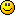#### Topic: Data import - Exclude Weekend Data

I modified the script to remove Saturday and Sunday candles.

``````for (int i = bars - 1; i >= 0; i--)
{
if (TimeDayOfWeek(Time[i]) != 0 && TimeDayOfWeek(Time[i]) != 6)
{
StringAdd(time,   IntegerToString((rates[i].time - millennium) / 60) + ",");
StringAdd(open,   IntegerToString((int) MathRound(rates[i].open  * multiplier)) + ",");
StringAdd(high,   IntegerToString((int) MathRound(rates[i].high  * multiplier)) + ",");
StringAdd(low,    IntegerToString((int) MathRound(rates[i].low   * multiplier)) + ",");
StringAdd(close,  IntegerToString((int) MathRound(rates[i].close * multiplier)) + ",");
StringAdd(volume, IntegerToString(rates[i].tick_volume) + ",");
}
}``````

Everything worked well!

Today I decided to update my data and now the script has stopped working, giving me array out of range error for that if-statement.

Only change was MT update, did it mess up the script behaviour?

#### Re: Data import - Exclude Weekend Data

The problem is that Time is the time of the current chart, but later you export rates ... Depending on the chart you run the script, it may happen that bars is longer than the number of bars on the current chart and Time[ i ] crashes.

The solution is simple - replace Time with rates[ i ].time:

``````    for (int i = bars - 1; i >= 0; i--)
{
int dayOfWeek = TimeDayOfWeek(rates[i].time);
if (dayOfWeek > SUNDAY && dayOfWeek < SATURDAY)
{
StringAdd(time,   IntegerToString((rates[i].time - millennium) / 60) + ",");
StringAdd(open,   IntegerToString((int) MathRound(rates[i].open  * multiplier)) + ",");
StringAdd(high,   IntegerToString((int) MathRound(rates[i].high  * multiplier)) + ",");
StringAdd(low,    IntegerToString((int) MathRound(rates[i].low   * multiplier)) + ",");
StringAdd(close,  IntegerToString((int) MathRound(rates[i].close * multiplier)) + ",");
StringAdd(volume, IntegerToString(rates[i].tick_volume) + ",");
}
}``````

..

and replacing the negation != with > <#### Re: Data import - Exclude Weekend DataIt works! Thank you, Miroslav, you're a star!

#### Re: Data import - Exclude Weekend Data

It might be for useful for others as well, data import script with weekend data exclusion below.

#### Re: Data import - Exclude Weekend Data

My 'secret' goal is to push EA Studio until I can net 3000 pips per day....

#### Re: Data import - Exclude Weekend Data

Yeah, dukas has weekends marked, very annoying. With this script no weekend bars.

#### Re: Data import - Exclude Weekend Data

Thanks.....!

So far, I have been using broker data (MT5).

Is there some reason why you have use dukas data instead of your broker.  Did you do some testing to see a benefit?

I have the script set to 100,000 bars.....

The data supplied by EA Studio is much less as Popov has tried to get quality data only.

I am kinda mixed up as to which to use.... broker data with errors or clean data supplied or. dukas. LOLOL

Lately I have been using the supplied data and been having good luck using the strategies.. of course, that could change as the weeks pass.

Opinion?

(I am running two copies to generate new strategies..... and a third to try to improve on the best strategies generated, like copying the generated strategy into the third copiy and adding a couple indicators to see if I can generate better quality metrics..... sorta tedious but getting some good results for very little effort because EA Studio is so powerful.)

My 'secret' goal is to push EA Studio until I can net 3000 pips per day....

#### Re: Data import - Exclude Weekend Data

As I'm working with 8 pairs, I need similar and same length datasets for proper evaluation and scenarios. That's why I went to duka data. Additionally, I can set up a bit higher spread with imported data, that way I make the test a bit harder.